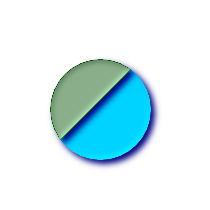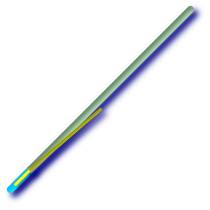## Name

ST_Split — Returns a collection of geometries resulting by splitting a geometry.

## Synopsis

`geometry ST_Split(`geometry input, geometry blade`)`;

## Description

The function supports splitting a line by point, a line by line, a polygon by line. The returned geometry is always a collection.

Think of this function as the opposite of ST_Union. Theoretically applying ST_Union to the elements of the returned collection should always yield the original geometry.

Availability: 2.0.0To improve the robustness of ST_Split it may be convenient to ST_Snap the input to the blade in advance using a very low tolerance. Otherwise the internally used coordinate grid may cause tolerance problems, where coordinates of input and blade do not fall onto each other and the input is not being split correctly (see #2192).

## Examples

Polygon Cut by LineBefore SplitAfter split
```-- this creates a geometry collection consisting of the 2 halves of the polygon
-- this is similar to the example we demonstrated in ST_BuildArea
SELECT ST_Split(circle, line)
FROM (SELECT
ST_MakeLine(ST_MakePoint(10, 10),ST_MakePoint(190, 190)) As line,
ST_Buffer(ST_GeomFromText('POINT(100 90)'), 50) As circle) As foo;

-- result --
GEOMETRYCOLLECTION(POLYGON((150 90,149.039264020162 80.2454838991936,146.193976625564 70.8658283817455,..), POLYGON(..)))

-- To convert to individual polygons, you can use ST_Dump or ST_GeometryN
SELECT ST_AsText((ST_Dump(ST_Split(circle, line))).geom) As wkt
FROM (SELECT
ST_MakeLine(ST_MakePoint(10, 10),ST_MakePoint(190, 190)) As line,
ST_Buffer(ST_GeomFromText('POINT(100 90)'), 50) As circle) As foo;

-- result --
wkt
---------------
POLYGON((150 90,149.039264020162 80.2454838991936,..))
POLYGON((60.1371179574584 60.1371179574584,58.4265193848728 62.2214883490198,53.8060233744357 ..))
```

Multilinestring Cut by pointBefore SplitAfter split
```SELECT ST_AsText(ST_Split(mline, pt)) As wktcut
FROM (SELECT
ST_GeomFromText('MULTILINESTRING((10 10, 190 190), (15 15, 30 30, 100 90))') As mline,
ST_Point(30,30) As pt) As foo;

wktcut
------
GEOMETRYCOLLECTION(
LINESTRING(10 10,30 30),
LINESTRING(30 30,190 190),
LINESTRING(15 15,30 30),
LINESTRING(30 30,100 90)
)
```